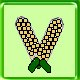Perl API

It is possible to write extensions to Webc in perl. These extensions are stored in files, and loaded in with the #localcode directive.

Functions can be called in a couple of ways. The currently preferred style is called from a webc file like:

```    #localcode calculate.lc
Calculate(3 * 4 + 5)
```

The localcode file might look something like:

```   #
#   calculate.lc
#
#   This defines the function Calculate which evaluates an
#   expression and returns the value.
#
use vars qw(%Callable);

sub Calculate
{
my(\$args) = @_;
my(\$result);

\$args =~ s/[^\d\-+*\/()]//g;
eval("\\$result = \$args");
return \$result;
}

\$Callable{'Calculate'} = 1;		# Let the function be called
return 1;
```
In this case, the string returned by the function is what is used in the webc file to replace the call.

There are a number of variables that you should know about. The %Symbols hash is the symbol table. Perl code is free to play with the symbols.

The OUT file handle is the current output stream (normally). Things written to this stream are put into the HTML output file.

[Introduction] [Overview] [Directives] [Variables] [Functions] [Invocation] [Suggestions] [Examples] [Perl API]
Source for this file can be found in api.wc.

[scripts] [Send a comment]